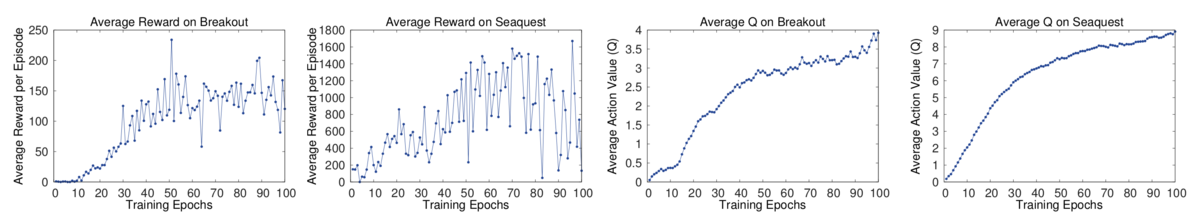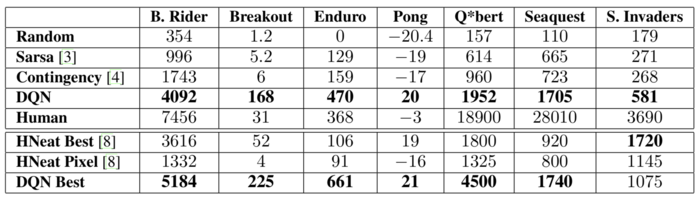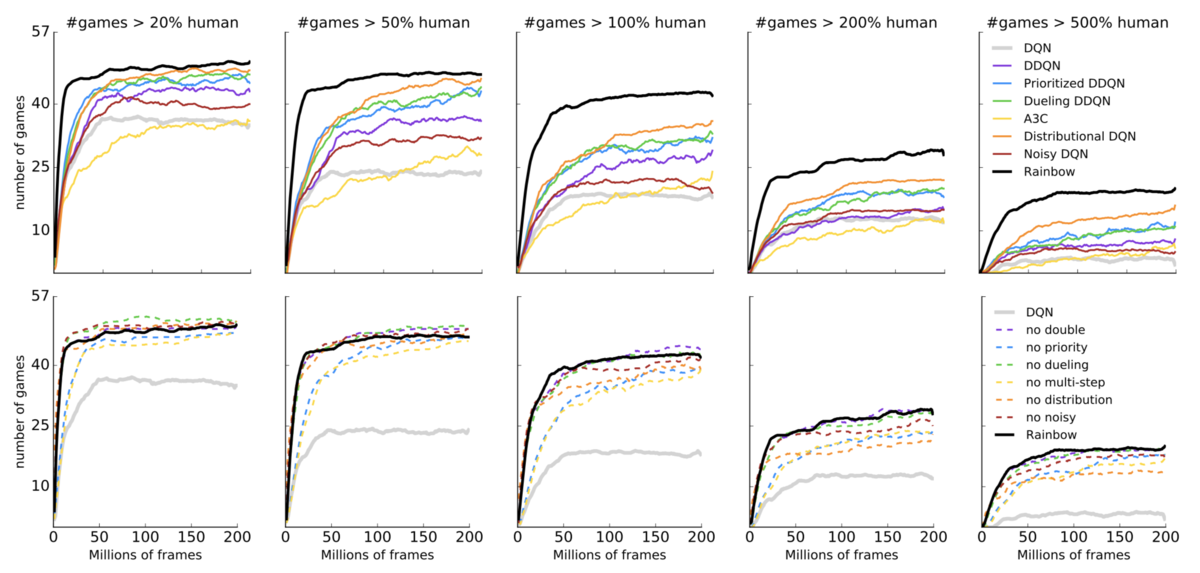# Course:CPSC522/Deep Reinforcement Learning

Jump to navigation Jump to search

## Deep Reinforcement Learning

Deep learning models such as neural networks that are used in reinforcement learning to learn control policies directly from high dimensional data are part of deep reinforcement learning. In other words, deep reinforcement learning is a combination of reinforcement learning and deep learning techniques.

Principal Author: Ainaz Hajimoradlou
Collaborators:

## Abstract

This page gives a short review of two papers in deep reinforcement learning. The first paper discusses a new approach for integrating deep learning techniques into reinforcement learning problems. The model is experimented on 7 different Atari games and is able to achieve higher scores in comparison to state of the art models at that time. The second paper expands this approach by 6 different techniques such as different architectures and sampling techniques on 57 Atari games. The model is able to achieve better scores compared to the previous approach.

### Builds on

This page builds on general concepts such as Deep Q-Learning and Neural Networks. The convolutional neural networks along with function approximators are used to model games like Alpha go using reinforcement learning techniques.

### Related Pages

Games like Alpha go are some examples of applications of deep reinforcement learning. These models are usually based on non-linear function approximators such as convolutional neural networks. Moreover, the essence of deep reinforcement learning algorithms is dependent on Deep Q-Learning.

## Content

### Paper I, Playing Atari with Deep Reinforcement Learning

#### Introduction

Throughout past years, deep learning techniques have been widely applied to many vision and speech problems and they were able to improve the final results to large extents. However, these techniques are not very successful when applied to reinforcement learning problems due to variety of reasons:

• Most of the successful deep learning techniques require a great amount of hand labelled data for training but reinforcement learning algorithms are trained based on a scalar reward which is usually noisy, sparse and delayed. In other words, in order to train an RL algorithm the corresponding reward for each possible action should be received which can take a long time (e.g. thousands of time steps). This is quite challenging compared to the direct relation between targets and inputs in supervised learning.
• Machine learning and deep learning methods are mostly relied on data being IID. However, most of the data sequences in reinforcement learning are highly correlated.
• Deep methods are trained based on the assumption that training/testing dataset has an unknown stationary distribution. But in reinforcement learning, distribution of the data changes whenever the model encounters new behaviours in the environment.

In order to alleviate these problems, experience replay techniques are often used in RL problems. This is typically sampling randomly from the previous experience or transitions. As a result, the training distribution becomes smoother and easier to learn.

This paper proposes a deep reinforcement learning algorithm for 7 different Atari games. No prior knowledge is passed to the agent except the possible actions, reward, terminal signals and the video screen of the play which is available to human players as well.

#### Background

The agent interacts with the environment $\varepsilon$in a sequence of actions, observations and rewards. Action $a_{t}$can be chosen from a set of legal actions $\mathrm {A} =\{1,...,K\}$. After doing an action, the internal state of the emulator along with the game score is changed. This change is the reward $r_{t}$at time step $t$. The environment can be stochastic or deterministic. As the agent is only able to observe the raw pixels of the screen and not the whole state, the task is partially observable. Therefore, in order to understand the current situation a sequence of actions and observations ($x_{1},a_{1},x_{2},a_{2},...,a_{t-1},x_{t}$) should be considered. Each sequence is a state ($s_{t}$) at time $t$. States are distinct and have the Markov property. Therefore, reinforcement learning techniques can be applied to this problem.

The goal is acting in a way that maximizes future rewards. But Instead of maximizing future rewards, future discounted return ($R_{t}=\sum _{t'=t}^{T}\gamma ^{t'-t}r_{t'}$) is maximized. $\gamma$is the discount factor and $T$is the finishing time step of the game. One of the reasons of using a discount factor is to avoid the sum of becoming infinite so that it can converge. Optimal action-value function $Q^{*}(s,a)$is defined as maximum expected return by following any strategy $\pi$, after seeing sate $s$and doing action $a$,

$Q^{*}(s,a)=\max _{\pi }\mathbb {E} \left[R_{t}|s_{t}=s,a_{t}=a,\pi \right].\quad (1)$This formula obeys Bellman equation. Meaning that, if the optimal value $Q^{*}(s',a')$of state $s'$is known at the next time step for all possible actions $a'$, the optimal strategy is choosing action $a'$that maximizes the expected value of $r+\gamma Q^{*}(s',a')$,

$Q^{*}(s,a)=\mathbb {E} _{s'\in \varepsilon }\left[r+\gamma \max _{a'}Q^{*}(s',a')|s,a\right].\quad (2)$Therefore, the basic idea of value iteration algorithms is to iteratively update the action-value function by using Bellman equation. This converges to the optimal action-value function as the iterations go to infinity. This is impractical because the function is estimated separately for each state, without generalization. In practice, a function approximator is used to estimate it which can be linear or nonlinear. Neural networks are nonlinear function approximators. If the parameters of the network is $\theta$, the loss function at each iteration $i$will be as follows.

$L_{i}(\theta _{i})=\mathbb {E} _{s,a\sim \rho (.)}\left[(y_{i}-Q(s,a;\theta _{i}))^{2}\right],\quad y_{i}=\mathbb {E} _{s'\sim \varepsilon }\left[r+\gamma \max _{a'}Q(s',a';\theta _{i-1})\right]\quad (3)$$y_{i}$is the target value for iteration $i$. The loss function simply tries to minimize the squared error between the predicted value of action-value function given current parameters and target value given previous parameters which is easily computed using bellman equation. $\rho (s,a)$is called the behaviour distribution and is simply the distribution over states and actions. The derivative of the loss function is as follows.

$\nabla L_{i}(\theta _{i})=\mathbb {E} _{s,a\sim \rho (.)|s'\sim \varepsilon }\left[\left(r+\gamma \max _{a'}Q(s',a';\theta _{i-1})-Q(s,a;\theta _{i})\right)\nabla _{\theta _{i}}Q(s,a;\theta _{i})\right]\quad (4)$In order to make gradient updates less computationally expensive, single samples from the behaviour distribution is used instead of using the full expectation. This distribution is often selected by an $\epsilon$-greedy approach. Moreover, the algorithm is model free and off policy.

#### Deep Reinforcement Learning

The goal of the paper is doing reinforcement learning using deep networks on Atari games without any preprocessing on the data. It's worth noting that combining model free reinforcement learning algorithms like Q-learning with non linear approximators or off policy learning can cause the network to diverge. Although this can partially be addressed by gradient temporal difference, the problem of using deep RL still remains a challenging problem.

In order to be able to use machine learning techniques on the problem, an experience replay technique should be used. This is simply storing the previous experiences $e_{t}=(s_{t},r_{t},s_{t+1})$into a replay memory ${\mathcal {D}}$. The pseudo code of the algorithm is shown below. In each time step of the game, Q-learning updates are applied to random samples of experiences stored in memory. After that, an action is chosen based on an $\epsilon$-greedy approach meaning that for probabilities below $\epsilon$a random action is chosen instead of the best possible action. $\phi$is a function that is used to produce fixed length representations for histories.

##### Deep Q-learning with experience replay
1. Initialize replay memory D to capacity N
2. Initialize action-value function Q with random weights
3. for episode = 1, M do
4.     Initialize sequence s1 = {x1} and preprocessed sequenced φ1 = φ(s1)
5.     for t = 1, T do
6.         With probability ε select a random action at
7.         otherwise select at = maxa Q∗(φ(st), a; θ)
8.         Execute action at in emulator and observe reward rt and image xt+1 Set st+1 = st, at, xt+1 and preprocess φt+1 = φ(st+1)
9.         Store transition (φt, at, rt, φt+1) in D
10.       Sample random minibatch of transitions (φj , aj , rj , φj +1 ) from D
11.       if φj+1 is terminal
12.           Set yj = rj
13.       else
14.           Set yj = rj + γ max_a′ Q(φj+1, a′; θ)
15.       Perform a gradient descent step on (yj − Q(φj , aj ; θ))^2
16.   end for
17. end for


This approach has three main advantages compared to approaches such as online Q-learning:

• It is more efficient as each step of experience is used in many weight updates.
• The updates are less variant as samples are chosen randomly instead of consecutively and this breaks the correlation between samples to some extent.
• In on-policy learning, the current parameters of the network determine the next data samples that the parameters should be trained on. As a result, if the maximizing action is to move left then data samples are dominated by the left hand side of the image. This will result in unwanted feedback loops or being stuck in local minima. Experience replay avoids such problems as behaviour distribution is averaged over its previous states, smoothing out learning and avoiding oscillations in parameters.

The proposed algorithm by the paper uses uniform sampling with a memory buffer of size $N$. However, this approach gives equal importance to all experiences and does not differentiate important transitions. A better sampling technique may be the one that puts more emphasis on transitions from which the model learns the most.

Training on the original pixel images of Atari is computationally expensive. Therefore, a preprocessing stage is used for resizing the images and converting them to grey scale. Besides, there are multiple ways to parametrize Q function using a neural network. This paper uses a convolutional architecture with only the state representation as input and multiple outputs corresponding to each action. Therefore, the network has one forward pass and Q values can be computed for all actions in each state. There are three hidden layers in the network and the final layer is a fully connected layer with a single output for each possible action.

#### Experiments

The model is trained with the same parameters for all different games. The only change is the scaling of the reward function: positive rewards are fixed to 1 and negative rewards are fixed to -1 with 0 being the unchanged rewards. Besides, the paper uses a frame-skipping technique that is instead of choosing actions in each frame, the actions are chosen every k frames and the last action is repeated in skipped frames. This will allow the agent to play roughly k times more without significantly increasing the runtime.Figure 1. Two figures on the left show the average reward per episode metric while the two on the right show the estimated Q function.

As with any machine learning algorithm, the results should be evaluated. Here, one reasonable choice might be tracking the average total reward metric. But, this tends to be very noisy as the small changes in weights of the network can result in big changes of the distribution. Therefore, a more stable metric can be the estimated value of the action-value function $Q$which shows how much discounted reward can be obtained by following a policy in a given state. Figure 1 shows the comparison between these two metrics for evaluation.Table 1. Comparing average total reward for different learning methods. Lower table shows the results obtained by different metrics of the proposed model with last row being the best results.

Table 1 shows different scores obtained by different methods on all the games. One of the scores is the one obtained by human players. The proposed model was able to achieve better results than humans in three of the games (Breakout, Enduro, Pong). Other than that, all final scores achieved by the model are higher than the state of the art methods on Atari games. One reason of getting lower scores than humans in some of the games may be the challenging nature of the game: finding a strategy that extends over long time scales.

#### Conclusion

Although obtaining results with deep reinforcement learning have proven to have low convergence rate, the model proposed in this paper was able to achieve state of the art results on Atari games by using experience replay techniques.

### Paper ||, Rainbow: Combining Improvements in Deep Reinforcement Learning

The main essence of this paper is based on an integrated method for extending the DQN algorithm, proposed in the previous paper. Generally, at each time step $t$the environment provides agent with an observation $S_{t}$. In response, the agent selects an action $A_{t}$which provides the next reward $R_{t+1}$, discount factor $\gamma _{t+1}$and state $S_{t+1}$. This can be formalized as a Markov Decision process $$. The members of the tuple correspond to the finite set of states, finite set of actions, transition function, reward function and the discount factor respectively. The action is chosen based on the policy $\pi$which is the distribution over actions. In DQN, parameters are optimized using the loss function proposed in equation (3). There are several limitations to this algorithm which are addressed by different methods. Some of these extensions are as follows:

• Double Q-learning: Learning in DQN is affected by an overestimation bias in the maximization step performed on loss function in equation (3). This can be addressed by decoupling the selection of the action from its evaluation. Therefore, the target value in the loss function will be changed as follows .
$y_{i}=\mathbb {E} _{s'\sim \varepsilon }\left[r+\gamma Q(s',\arg \max _{a'}Q(s',a';\theta _{i-1});\theta _{i-1})\right]$• Prioritized replay: In DQN, the samples are chosen uniformly from the replay memory. However, the samples from which the network learns the most should be chosen more frequently. The idea is using prioritized experience replay. Therefore, transitions that are relevant to the last encountered TD error will have higher probabilities and are chosen most of the times.
• Dueling networks: This is a neural network architecture which is designed for value based reinforcement learning. So, instead of using a convolutional neural network to approximate action-value function in DQN, a shared convolutional encoder along with a special aggregator is used.
• Multi-step Learning: DQN accumulates a single reward and then uses greedy action at the next step to bootstrap. An an alternative, forward-view multi-step targets can be used for optimizing the parameters which can lead to faster learning with suitable $n$. Therefore, the reward function will be defined as follows.
$R_{t}^{(n)}\equiv \sum _{k=0}^{n-1}\gamma _{t}^{(k)}R_{t+k+1}$• Distributional RL: Instead of approximating the expected return, the distribution of returns can be estimated. Therefore, the output of the network would be the distribution over each action.
• Noisy Nets: Exploration using $\epsilon$-greedy approaches is not suitable for situations where a lot of actions should be executed to collect the first reward. In these cases a model with a linear layer composed of a deterministic and noisy stream is proposed. The model makes the network learn that it can ignore the noisy stream. The extent varies as the state space changes. This will allow state-conditional exploration with a form of self annealing. $\epsilon ^{b}$and $\epsilon ^{w}$are random variables and $\odot$is the element-wise multiplication.
$y=(b+Wx)+(b_{noisy}\odot \epsilon ^{b}+(W_{noisy}\odot \epsilon ^{w})x)$The paper uses the integrated version of all above extensions as the original model. They combine a multi-step distributional loss with double Q-learning to choose the best action. They use prioritized experience replay to store the most frequent transitions in the memory. The network architecture is a dueling network which is adapted to use return distributions and all the linear layers are replaced with their equivalent noisy nets.

#### Experiments

The model is evaluated on 57 Atari games. The results are compared to human performance in all games. As the model is actually a combination of 6 other models, the number of hyper-parameters is large. Therefore, some tuning is needed for each component. The initial values are the values proposed by the original papers corresponding to each component. Then, the most sensitive ones are tuned using manual coordinate descent. The hyper parameters are the same across all games.Figure 2. First row shows the number of games where each agent was able to achieve at least a fraction of human performance as a function of time. The bottom row compares performance of rainbow to the models where some component is removed from the original model.

Figure 2, top row, shows different plots for several agents, each plot represents the number of games the agent was able to achieve a given fraction of human performance. Bottom row shows the significance of each component in the integrated model by removing each component from the model and observing the performance.

### Discussion

The first paper introduces a new approach to deep reinforcement learning by a non-linear function approximator. Although, the chances of getting a deep network to work with reinforcement learning algorithms is very low, the paper was able to achieve not only reasonably good results but higher scores compared to the state of the art approaches which was a big breakthrough in this area. On the other hand, the second paper is actually just an integration method of different existing extensions to the proposed algorithm in the first paper. Each of these extensions have been used to improve one of the limitations of deep Q-learning. Therefore, having a model composed of all these extensions has resulted in improvement of the original model.

## Annotated Bibliography

Put your annotated bibliography here. Add links where appropriate.

1. V. Minh, et al., Playing Atari with Deep Reinforcement Learning
2. Hado van Hasselt, Arthur Guez, David Silver, Deep Reinforcement Learning with Double Q-learning
3. Tom Schaul, John Quan, Ioannis Antonoglou, and David Silver, Prioritized Experience Replay
4. Ziyu Wang, Tom Schaul, Matteo Hessel, Hado van Hasselt, Marc Lanctot and Nando de Freitas, Dueling Network Architectures for Deep Reinforcement Learning
5. Richard S Sutton, Learning to predict by the methods of temporal differences
6. Bellemare, Dabney, and Munos, Distributional Reinforcement Learning with Quantile Regression
7. Fortunato et. al., Noisy Networks for Exploration
8. M. Hessel, et. al., Rainbow: Combining Improvements in Deep Reinforcement LearningPermission is granted to copy, distribute and/or modify this document according to the terms in Creative Commons License, Attribution-NonCommercial-ShareAlike 3.0. The full text of this license may be found here: CC by-nc-sa 3.0